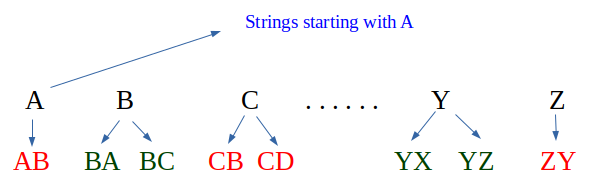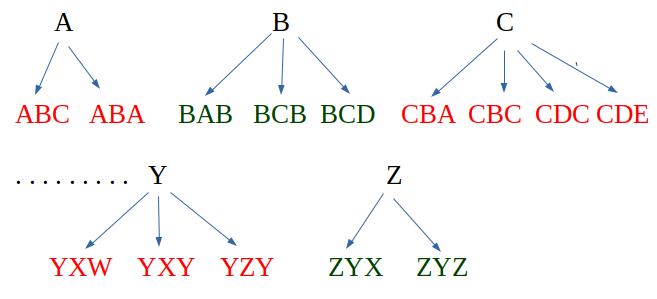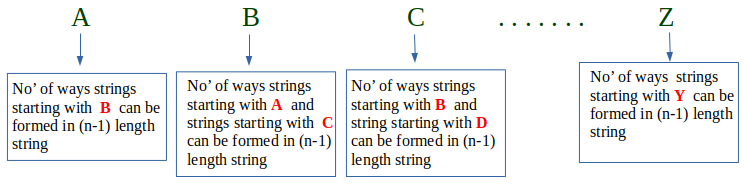# Count of strings where adjacent characters are of difference one

iven a number n, count number of strings of length n such that every string has adjacent characters with difference between ASCII values as 1.

Examples:

```Input :  N = 1
Output : Total strings are 26
Explanation : For N=1, strings
are a, b, c,, ...., x, y, z

Input :  N = 2
Output : Total strings are 50
Explanation : For N = 2, strings
are ab, ba, bc, cb, .., yx, yz, zy
```

## Recommended: Please try your approach on {IDE} first, before moving on to the solution.

For strings starting with character ‘A’ and length ‘i’, we consider all strings of length ‘i-1’ and starting with character ‘B’

For strings starting with character ‘G’ and length ‘i’, we consider all strings of length ‘i-1’ and starting with character ‘H’ and all strings of length ‘i-1’ and starting with ‘F’.

We take the base case for n = 1, and set result for all 26 characters as 1. This simply means when 1 character string is consider all alphabets from a-z are taken only once.

For N = 2,For N = 3,Conclusion : For N = n```countAdjacent(n)
dp[i][j] finally stores count of strings
of length i and starting with
character j.

Initialize dp[n+1] as 0
Initialize dp[j] = 1 where j = 0 to 25
for i = 2 to n
for j = 0 to 25
if (j = 0)
dp[i][j] = dp[i-1][j+1];
else
dp[i][j] = dp[i-1][j-1] + dp[i-1][j+1];
Sum of n-th row from 0 to 25 is the result.
```

## C++

 `// CPP Program to count strings with adjacent ` `// characters. ` `#include ` `using` `namespace` `std; ` ` `  `int` `countStrs(``int` `n) ` `{ ` `    ``long` `int` `dp[n + 1]; ` ` `  `    ``// Initializing arr[n+1] to 0 ` `    ``memset``(dp, 0, ``sizeof``(dp)); ` ` `  `    ``// Initialing 1st row all 1 from 0 to 25 ` `    ``for` `(``int` `i = 0; i <= 25; i++) ` `        ``dp[i] = 1; ` ` `  `    ``// Begin evaluating from i=2 since 1st row is set ` `    ``for` `(``int` `i = 2; i <= n; i++) { ` `        ``for` `(``int` `j = 0; j <= 25; j++) ` ` `  `            ``// j=0 is 'A' which can make strings  ` `            ``// of length i using strings of length  ` `            ``// i-1 and starting with 'B' ` `            ``if` `(j == 0)  ` `                ``dp[i][j] = dp[i - 1][j + 1]; ` `            ``else` `                ``dp[i][j] = (dp[i - 1][j - 1] + ` `                            ``dp[i - 1][j + 1]); ` `    ``} ` ` `  `    ``// Our result is sum of last row. ` `    ``long` `int` `sum = 0; ` `    ``for` `(``int` `i = 0; i <= 25; i++) ` `        ``sum = (sum + dp[n][i]); ` `    ``return` `sum; ` `} ` ` `  `// Driver's Code ` `int` `main() ` `{ ` `    ``int` `n = 3; ` `    ``cout << ``"Total strings are : "` `<< countStrs(n); ` `    ``return` `0; ` `} `

## Java

 `// Java Program to count strings  ` `// with adjacent characters.  ` `class` `GFG  ` `{ ` ` `  `    ``static` `long` `countStrs(``int` `n)  ` `    ``{ ` `        ``long``[][] dp = ``new` `long``[n + ``1``][``27``]; ` ` `  `        ``// Initializing arr[n+1] to 0  ` `        ``for` `(``int` `i = ``0``; i < n + ``1``; i++)  ` `        ``{ ` `            ``for` `(``int` `j = ``0``; j < ``27``; j++) ` `            ``{ ` `                ``dp[i][j] = ``0``; ` `            ``} ` `        ``} ` ` `  `        ``// Initialing 1st row all 1 from 0 to 25  ` `        ``for` `(``int` `i = ``0``; i <= ``25``; i++) ` `        ``{ ` `            ``dp[``1``][i] = ``1``; ` `        ``} ` ` `  `        ``// Begin evaluating from i=2  ` `        ``// since 1st row is set  ` `        ``for` `(``int` `i = ``2``; i <= n; i++)  ` `        ``{ ` `             `  `            ``// j=0 is 'A' which can make strings ` `            ``for` `(``int` `j = ``0``; j <= ``25``; j++)   ` `             `  `            ``// of length i using strings of length  ` `            ``// i-1 and starting with 'B'  ` `            ``{ ` `                ``if` `(j == ``0``)  ` `                ``{ ` `                    ``dp[i][j] = dp[i - ``1``][j + ``1``]; ` `                ``}  ` `                ``else`  `                ``{ ` `                    ``dp[i][j] = (dp[i - ``1``][j - ``1``] ` `                            ``+ dp[i - ``1``][j + ``1``]); ` `                ``} ` `            ``} ` `        ``} ` ` `  `        ``// Our result is sum of last row.  ` `        ``long` `sum = ``0``; ` `        ``for` `(``int` `i = ``0``; i <= ``25``; i++)  ` `        ``{ ` `            ``sum = (sum + dp[n][i]); ` `        ``} ` `        ``return` `sum; ` `    ``} ` ` `  `    ``// Driver Code ` `    ``public` `static` `void` `main(String[] args) ` `    ``{ ` `        ``int` `n = ``3``; ` `        ``System.out.println(``"Total strings are : "` `+ ` `                                        ``countStrs(n)); ` `    ``} ` `} ` ` `  `// This code is contributed by 29AjayKumar `

## Python 3

 `# Python3 Program to count strings with ` `# adjacent characters.  ` `def` `countStrs(n): ` ` `  `    ``# Initializing arr[n+1] to 0  ` `    ``dp ``=` `[[``0` `for` `j ``in` `range``(``27``)]  ` `             ``for` `i ``in` `range``(n ``+` `1``)] ` ` `  `    ``# Initialing 1st row all 1 from 0 to 25 ` `    ``for` `i ``in` `range``(``0``, ``26``): ` `        ``dp[``1``][i] ``=` `1` ` `  `    ``# Begin evaluating from i=2 since  ` `    ``# 1st row is set      ` `    ``for` `i ``in` `range``(``2``, n ``+` `1``): ` `        ``for` `j ``in` `range``(``0``, ``26``): ` ` `  `            ``# j=0 is 'A' which can make strings  ` `            ``# of length i using strings of length  ` `            ``# i-1 and starting with 'B'  ` `            ``if``(j ``=``=` `0``): ` `                ``dp[i][j] ``=` `dp[i ``-` `1``][j ``+` `1``]; ` `            ``else``: ` `                ``dp[i][j] ``=` `(dp[i ``-` `1``][j ``-` `1``] ``+` `                            ``dp[i ``-` `1``][j ``+` `1``]) ` ` `  `    ``# Our result is sum of last row.          ` `    ``sum` `=` `0` `    ``for` `i ``in` `range``(``0``, ``26``): ` `        ``sum` `=` `sum` `+` `dp[n][i] ` ` `  `    ``return` `sum` `     `  `# Driver's Code  ` `if` `__name__ ``=``=` `"__main__"``: ` `    ``n ``=` `3` `    ``print``(``"Total strings are : "``, countStrs(n)) ` `     `  `# This code is contributed by Sairahul Jella `

## C#

 `// C# Program to count strings with   ` `// adjacent characters.  ` `using` `System;  ` ` `  `class` `GFG  ` `{  ` `    ``static` `long` `countStrs(``int` `n)  ` `    ``{  ` `        ``long``[,] dp = ``new` `long``[n + 1, 27];  ` `     `  `        ``// Initializing arr[n+1] to 0  ` `        ``for``(``int` `i = 0; i < n + 1; i++) ` `            ``for``(``int` `j = 0; j < 27; j++) ` `                ``dp[i, j] = 0; ` `     `  `        ``// Initialing 1st row all 1 from 0 to 25  ` `        ``for` `(``int` `i = 0; i <= 25; i++)  ` `            ``dp[1, i] = 1;  ` `     `  `        ``// Begin evaluating from i=2 since 1st row is set  ` `        ``for` `(``int` `i = 2; i <= n; i++) ` `        ``{  ` `            ``for` `(``int` `j = 0; j <= 25; j++)  ` `     `  `                ``// j=0 is 'A' which can make strings  ` `                ``// of length i using strings of length  ` `                ``// i-1 and starting with 'B'  ` `                ``if` `(j == 0)  ` `                    ``dp[i, j] = dp[i - 1, j + 1];  ` `                ``else` `                    ``dp[i, j] = (dp[i - 1, j - 1] +  ` `                                ``dp[i - 1, j + 1]);  ` `        ``}  ` `     `  `        ``// Our result is sum of last row.  ` `        ``long` `sum = 0;  ` `        ``for` `(``int` `i = 0; i <= 25; i++)  ` `            ``sum = (sum + dp[n, i]);  ` `        ``return` `sum;  ` `    ``}  ` `     `  `    ``// Driver Code ` `    ``static` `void` `Main()  ` `    ``{  ` `        ``int` `n = 3;  ` `        ``Console.Write(``"Total strings are : "` `+ countStrs(n));  ` `    ``} ` `} ` ` `  `// This code is contributed by DrRoot_ `

Output:

```Total strings are : 98
```

This article is contributed by Shubham Rana. If you like GeeksforGeeks and would like to contribute, you can also write an article using contribute.geeksforgeeks.org or mail your article to contribute@geeksforgeeks.org. See your article appearing on the GeeksforGeeks main page and help other Geeks.